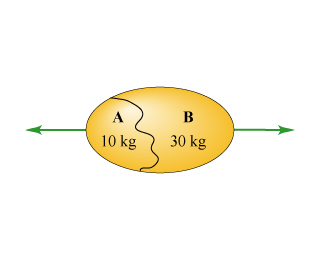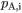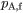# Problem: A giant "egg" explodes as part of a fireworks display. The egg is at rest before the explosion, and after the explosion, it breaks into two pieces, with the masses indicated in the diagram, traveling in opposite directions.Part AWhat is the momentum  of piece A before the explosion?Express your answer numerically in kilogram meters per second.Part BDuring the explosion, is the force of piece A on piece B greater than, less than, or equal to the force of piece on piece A?Part CThe momentum of piece B is measured to be 500 kg•m/s after the explosion. Find the momentum of piece A after the explosion.Enter your answer numerically in kilogram meters per second.

###### FREE Expert Solution

Momentum,

$\overline{){\mathbf{P}}{\mathbf{=}}{\mathbf{m}}{\mathbf{v}}}$

Part A

vA,i = 0

89% (362 ratings)###### Problem Details

A giant "egg" explodes as part of a fireworks display. The egg is at rest before the explosion, and after the explosion, it breaks into two pieces, with the masses indicated in the diagram, traveling in opposite directions.Part A

What is the momentumof piece A before the explosion?

Express your answer numerically in kilogram meters per second.

Part B

During the explosion, is the force of piece A on piece B greater than, less than, or equal to the force of piece on piece A?

Part C

The momentum of piece B is measured to be 500 kg•m/s after the explosion. Find the momentumof piece A after the explosion.

Enter your answer numerically in kilogram meters per second.

Frequently Asked Questions

What scientific concept do you need to know in order to solve this problem?

Our tutors have indicated that to solve this problem you will need to apply the Push-Away Problems With Energy concept. You can view video lessons to learn Push-Away Problems With Energy. Or if you need more Push-Away Problems With Energy practice, you can also practice Push-Away Problems With Energy practice problems.

What professor is this problem relevant for?

Based on our data, we think this problem is relevant for Professor Bergeler's class at TXSTATE.